﻿ 用预期寿命预测中国未来人口死亡率

# 用预期寿命预测中国未来人口死亡率Using Life Expectancy to Predict China’s Future Population Mortality

Abstract: There is a high correlation between the life expectancy of the population and the mortality rate. The LL (Liner-Link) model measures this correlation and improves the prediction accuracy. This paper compares the five binomial mortality models with the LL model. The LL model is tested based on the mortality data of Taiwan’s population. The historical mortality data of the mainland population is used to estimate the parameters and predict the future mortality trends. And the mortality rate improvement level was measured, it was found that the mortality rate improved over time.

1. 引言

LC系模型(Lee-Carter模型 、Renshaw-Haberman模型  和Age-Period-Cohort模型  )和CBD系模型  在人口统计学和寿险精算文献中很常见，前者假设分年龄死亡人数服从泊松分布，基于对数变换后的中心死亡率进行建模；后者假设分年龄死亡人数服从二项分布，基于log it变换后的死亡概率进行建模。在本文中我们对LC系模型和PLAT模型  也使用log it变换，然后将这五种二项式死亡率模型与LL模型进行对比研究。

2. 数据和方法

2.1. 数据选取

2.2. 二项式死亡率模型Table 1. Binomial mortality prediction model

2.3. LL模型

Pascariu等(2020)  通过研究发现预期寿命取对数后和对数中心死亡率之间存在线性关系并可用如下关系式表示：

$\mathrm{log}{m}_{x,t}={\beta }_{x}\mathrm{log}{e}_{\theta ,t}+{\epsilon }_{x,t}$ (1)

$\mathrm{log}{m}_{x,t}={\beta }_{x}\mathrm{log}{e}_{\theta ,t}+{v}_{x}k+{\epsilon }_{x,t}$ (2)

2.4. 死亡率年均改善指标

Purushotham等(2011)  采用死亡率年均改善指标对死亡率改善水平进行度量，定义如下：

${r}_{x}^{t~t+k}=1-{\left(\frac{{q}_{x}^{t+k}}{{q}_{x}^{t}}\right)}^{\frac{1}{k}}$ (3)

3. 对比分析

3.1. 模型检验Table 2. Pearson correlation coefficient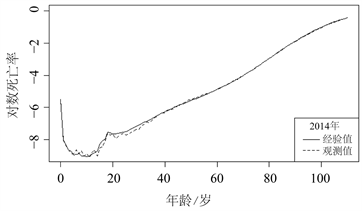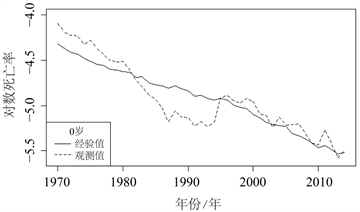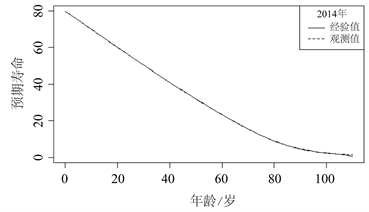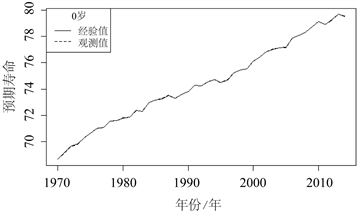Figure 1. Comparison of Taiwan’s empirical observations

3.2. 模型对比

3.2.1. 拟合效果检验Table 3. Comparison of binomial mortality models

3.2.2. 参数估计

(1) 计观察时间 $t$ 内预期寿命和死亡率之间的线性关系的斜率 ${\beta }_{x}$ ：通过使用最小二乘法并最小化残差平方

$\underset{x}{\sum }{\left[\mathrm{log}{m}_{x,t}-{\beta }_{x}\mathrm{log}{e}_{\theta ,t}\right]}^{2}=\underset{x}{\sum }{\left[{\epsilon }_{x,t}\right]}^{2}$ (4)

(2) 通过计算回归残差矩阵R的奇异值分解(SVD)来估计参数 ${v}_{x}$

$SVD\left[R\right]=DP{Q}^{T}={d}_{1}{p}_{1}{q}_{1}^{T}$

$R=\left(\begin{array}{cccc}{\epsilon }_{0,1}& {\epsilon }_{0,2}& \cdots & {\epsilon }_{0,T}\\ {\epsilon }_{1,1}& {\epsilon }_{1,2}& \cdots & {\epsilon }_{1,T}\\ ⋮& ⋮& \ddots & ⋮\\ {\epsilon }_{w,0}& {\epsilon }_{w,1}& \cdots & {\epsilon }_{w,T}\end{array}\right)$

$P=\left[{p}_{1},{p}_{2},\cdots \right]$$Q=\left[{q}_{1},{q}_{2},\cdots \right]$ 是左右奇异向量的矩阵，D是奇异值沿对角线的对角矩阵。SVD的第一项 ${d}_{1}{p}_{1}{q}_{1}^{T}$ 用于获得 ${v}_{x}$ 的估计值。

(3) 使用样条平滑参数 ${\beta }_{x}$${v}_{x}$，并使用估计校正系数 $k$ 对死亡率曲线进行优化。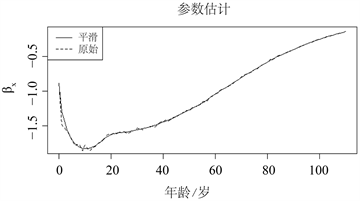Figure 2. LL model parameter estimation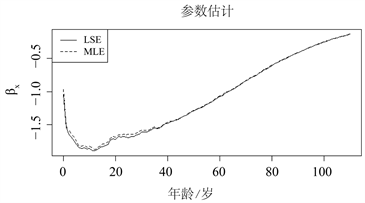Figure 3. Use LSE and MLE to estimate the parameters of the LL model

3.2.3. 样本外数据预测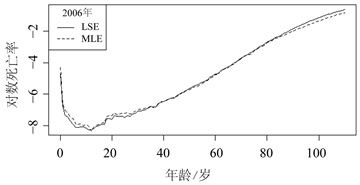Figure 4. Use LSE and MLE to fit logarithmic mortality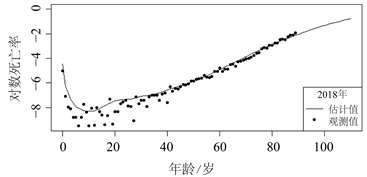Figure 5. Observed and estimated log mortality of the mainland population

3.2.4. 预测

(1) 利用LL模型使用1995~2018年的历史数据在0~89岁年龄范围内进行拟合，并用于预测未来22年直至2040年的死亡率；

(2) 使用Kannisto模型将中国0~89岁之间的观测死亡率矩阵扩展至110岁，方法：用80~89岁的数据进行拟合，预测90~110岁的数据；

(3) 一种模型如果对同一个预测点模拟多个预测，将产生一系列结果，这些结果可通过标准寿命表计算转化为预期寿命；

(4) 由LC模型给出的任何预测预期寿命值都被用作LL模型中的输入值，以导出死亡率曲线，从而获得两条可比较的曲线；

(5) 对于每个模拟的轨迹，LL方法可以产生死亡率曲线，产生中值预测的不确定性；

(6) 99%的预测区间是基于一千次蒙特卡罗模拟计算的。Table 5. Coefficient predicted value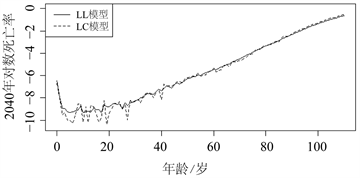Figure 6. Comparison of logarithmic mortality curves predicted by LL model and LC model

4. 死亡率改善水平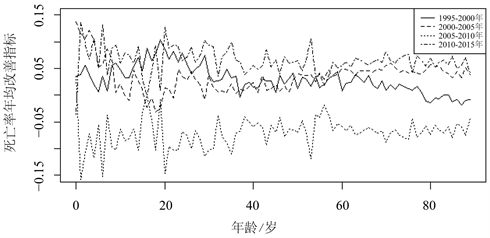Figure 7. Continental observations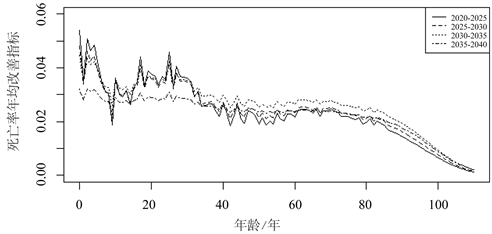Figure 8. Continental predicted value

5. 结论

 王晓军, 路倩. 动态死亡率模型的研究进展[J]. 应用概率统计, 2020, 36(4): 415-440.

 王晓军, 米海杰. 中国人口死亡率改善水平比较分析[J]. 统计研究, 2013, 30(2): 58-63.

 曾燕, 陈曦, 邓颖璐. 创新的动态人口死亡率预测及其应用[J]. 系统工程理论与实践, 2016, 36(7): 1710-1718.

 胡仕强, 陈荣达. 我国有限人口数据下长寿衍生产品定价的贝叶斯方法[J]. 系统科学与数学, 2018, 38(4): 497-510.

 陈宁. 基于神经网络死亡率模型的我国死亡率预测及应用[D]: [硕士学位论文]. 天津: 天津财经大学, 2019.

 Pascariu, M.D., Basellini, U., Aburto, J.M. and Canudas-Romo, V. (2020) The Linear Link: Deriving Age-Specific Death Rates from Life Expectancy. Risks, 8, 109-126.
https://doi.org/10.3390/risks8040109

 Lee, R.D. and Carter, L.R. (1992) Modeling and Forecasting U.S. Mortality. Journal of the American Statistical Association, 87, 659-671.
https://doi.org/10.1080/01621459.1992.10475265

 Renshaw, A.E., Haberman, S. (2006) A Cohort-Based Extension to the Lee-Carter Model for Mortality Reduction Factors. Insurance: Mathematics and Economics, 38, 556-570.
https://doi.org/10.1016/j.insmatheco.2005.12.001

 Currie, I.D., Durban, M. and Eilers, P.H.C. (2006) Generalized Linear Array Models with Applications to Multidimensional Smoothing. Journal of the Royal Statistical Society, 68, 259-280.
https://doi.org/10.1111/j.1467-9868.2006.00543.x

 Cairns, A.J.G., Blake, D. and Dowd, K. (2006) A Two-Factor Model for Stochastic Mortality with Parameter Uncertainty: Theory and Calibration. Journal of Risk and Insurance, 73, 687-718.
https://doi.org/10.1111/j.1539-6975.2006.00195.x

 Plat, R. (2009) On Stochastic Mortality Modelling. Insurance: Mathematics and Economics, 45, 393-404.
https://doi.org/10.1016/j.insmatheco.2009.08.006

 Purushotham, M., Valdez, E. and Wu, H. (2011) Global Mortality Improvement Experience and Projection Techniques. Society of Actuaries.

 Thatcher, A.R., Kannisto, V. and Vaupl, J.W. (1998) The Force of Mortality at Ages 80 to 120. Odense University Press, Odense.

Top